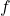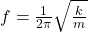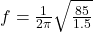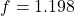## A 1.5kg mass attached to an ideal spring oscillates horizontally with an amplitude of 0.50m. The spring constant is 85 n/m. what is the freq

Question

A 1.5kg mass attached to an ideal spring oscillates horizontally with an amplitude of 0.50m. The spring constant is 85 n/m. what is the frequency of the mass’s motion?

in progress 0
3 months 2021-07-25T02:41:52+00:00 1 Answers 0 views 0

frequency of the mass motion≅ 1.2 Hz

Explanation:

Given that:

Mass m = 1.5 kg

Amplitude A = 0.50 m

Spring constant k = 85 n/m

The frequency can be calculated by using the formula:Hz

frequency of the mass motion≅ 1.2 Hz